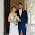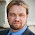Skip to main content

### What's the Value of a Win?

In a previous entry I demonstrated one simple way to estimate an exponent for the Pythagorean win expectation. Another nice consequence of a Pythagorean win expectation formula is that it also makes it simple to estimate the run value of a win in baseball, the point value of a win in basketball, the goal value of a win in hockey etc.

Let our Pythagorean win expectation formula be $w=\frac{P^e}{P^e+1},$ where $$w$$ is the win fraction expectation, $$P$$ is runs/allowed (or similar) and $$e$$ is the Pythagorean exponent. How do we get an estimate for the run value of a win? The expected number of games won in a season with $$g$$ games is $W = g\cdot w = g\cdot \frac{P^e}{P^e+1},$ so for one estimate we only need to compute the value of the partial derivative $$\frac{\partial W}{\partial P}$$ at $$P=1$$. Note that $W = g\left( 1-\frac{1}{P^e+1}\right),$ and so $\frac{\partial W}{\partial P} = g\frac{eP^{e-1}}{(P^e+1)^2}$ and it follows $\frac{\partial W}{\partial P}(P=1) = \frac{ge}{4}.$ Our estimate for the run value of a win now follows by setting $\frac{\Delta W}{\Delta P} = \frac{ge}{4}$ giving $\Delta W = 1 = \frac{ge}{4} \Delta P.$ What is $$\Delta P$$? Well $$P = R/A$$, where $$R$$ is runs scored over the season and $$A$$ is runs allowed over the season. We're assuming this is a league average team and asking how many more runs they'd need to score to win an additional game, so $$A$$ is actually fixed at $$L$$, the league average number of runs scored (or allowed). This gives us $1 = \frac{ge}{4} \Delta P = \frac{ge\Delta R}{4L}.$ Now $$L/g = l$$, the league average runs per game, so we arrive at the estimate $\Delta R = \frac{4l}{e}.$ In the specific case of MLB, we have $$e = 1.8$$ and $$l = 4.3$$, giving that a win is approximately $$\Delta R = 9.56$$ runs.

Bill James originally used the exponent $$e=2$$; in this case the formula simplifies to $$\Delta R = 2l$$, i.e. we get the particularly simple result that a win is equal to approximately twice the average number of runs scored per game.

Applying this estimate to the NBA, a win is approximately $$\Delta R = \frac{4\cdot 101}{16.4} = 24.6$$ points. Similarly, we get the estimates for a win of 4.5 goals for the NHL and 5.1 goals for the Premier League.

### Comments

1.I think you've assigned the incorrect goals/win to the wrong league. NHL I think is 5.1 and Premier League is 4.5. Thanks for sharing!!

2.Thanks, I believe you're right!

### A Bayes' Solution to Monty Hall

For any problem involving conditional probabilities one of your greatest allies is Bayes' Theorem. Bayes' Theorem says that for two events A and B, the probability of A given B is related to the probability of B given A in a specific way.

Standard notation:

probability of A given B is written $$\Pr(A \mid B)$$
probability of B is written $$\Pr(B)$$

Bayes' Theorem:

Using the notation above, Bayes' Theorem can be written: $\Pr(A \mid B) = \frac{\Pr(B \mid A)\times \Pr(A)}{\Pr(B)}$Let's apply Bayes' Theorem to the Monty Hall problem. If you recall, we're told that behind three doors there are two goats and one car, all randomly placed. We initially choose a door, and then Monty, who knows what's behind the doors, always shows us a goat behind one of the remaining doors. He can always do this as there are two goats; if we chose the car initially, Monty picks one of the two doors with a goat behind it at random.

Assume we pick Door 1 and then Monty sho…

### Mixed Models in R - Bigger, Faster, Stronger

When you start doing more advanced sports analytics you'll eventually starting working with what are known as hierarchical, nested or mixed effects models. These are models that contain both fixed and random effects. There are multiple ways of defining fixed vs random random effects, but one way I find particularly useful is that random effects are being "predicted" rather than "estimated", and this in turn involves some "shrinkage" towards the mean.

Here's some R code for NCAA ice hockey power rankings using a nested Poisson model (which can be found in my hockey GitHub repository):
model <- gs ~ year+field+d_div+o_div+game_length+(1|offense)+(1|defense)+(1|game_id) fit <- glmer(model, data=g, verbose=TRUE, family=poisson(link=log) ) The fixed effects are year, field (home/away/neutral), d_div (NCAA division of the defense), o_div (NCAA division of the offense) and game_length (number of overtime periods); off…

### Notes on Setting up a Titan V under Ubuntu 17.04

I recently purchased a Titan V GPU to use for machine and deep learning, and in the process of installing the latest Nvidia driver's hosed my Ubuntu 16.04 install. I was overdue for a fresh install of Linux, anyway, so I decided to upgrade some of my drives at the same time. Here are some of my notes for the process I went through to get the Titan V working perfectly with TensorFlow 1.5 under Ubuntu 17.04.

Old install:
Ubuntu 16.04
EVGA GeForce GTX Titan SuperClocked 6GB
2TB Seagate NAS HDD
+ additional drives

New install:
Ubuntu 17.04
Titan V 12GB
/ partition on a 250GB Samsung 840 Pro SSD (had an extra around)
/home partition on a new 1TB Crucial MX500 SSD
New WD Blue 4TB HDD
+ additional drives

You'll need to install Linux in legacy mode, not UEFI, in order to use Nvidia's proprietary drivers for the Titan V. Note that Linux will cheerfully boot in UEFI mode, but will not load any proprietary drivers (including Nvidia's). You'll need proprietary drivers for Tens…